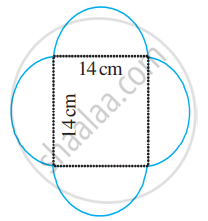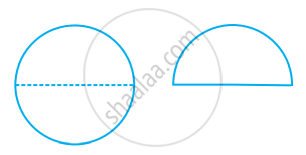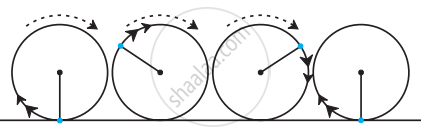# Circumference of a Circle

## Definition

Circumference of a circle: The circumference or the perimeter of a circle refers to the measurement of the border across any 2D circular shape including the circle.

## Formula

Circumference of a circle = 2πr

## Notes

### Circumcircle or Perimeter of a circle:

• The word perimeter means a path that surrounds an area.

• It comes from the Greek word 'peri,' meaning around, and 'metron,' which means measure.

• Perimeter can be defined as the path or the boundary that surrounds a shape. It can also be defined as the length of the outline of a shape.

• The circumference or the perimeter of a circle refers to the measurement of the border across any 2D circular shape including the circle.

• Circumference of a circle bears a constant ratio with its diameter. This constant ratio is denoted by the Greek letter π (read as ‘pi’).
In other words,

"circumference"/"diameter" = π

or, circumference = π × diameter
= π × 2r....................(where r is the radius of the circle)
= 2πr.

π is an irrational number and its decimal expansion is non-terminating and non-recurring (non-repeating).

However, for practical purposes, we generally take the value of π as 22/7 or 3.14, approximately.

## Example

What is the circumference of a circle of diameter 10 cm (Take π = 3.14)?

Diameter of the circle (d) = 10 cm

Circumference of circle = πd

= 3.14 × 10 cm

= 31.4 cm

So, the circumference of the circle of diameter 10 cm is 31.4 cm.

## Example

Find the perimeter of the given shape (Take π = 22/7).The outer boundary, of this figure, is made up of semicircles.
Diameter of each semicircle is 14 cm.

We know that:
Circumference of the circle = πd
Circumference of the semicircle = 1/2πd

= 1/2 xx 22/7 × 14 cm

= 22 cm

Circumference of each of the semicircles is 22 cm

Therefore, perimeter of the given figure = 4 × 22 cm = 88 cm.

## Example

Sudhanshu divides a circular disc of radius 7 cm in two equal parts. What is the perimeter of each semicircular shape disc? (Use π = 22/7)Given that radius (r) = 7 cm.
We know that the circumference of circle = 2πr

So, the circumference of the semicircle = 1/2 × 2πr = πr

= 22/7 × 7 cm

= 22 cm

So, the diameter of the circle =2r = 2 × 7 cm = 14 cm

Thus, perimeter of each semicircular disc = 22 cm + 14 cm = 36 cm.

## Example

The radius of a circular plot is 7.7 metres. How much will it cost to fence the plot with 3 rounds of wire at the rate of 50 rupees per metre?

Circumference of circular plot = 2πr = 2 xx 22/7 xx 7.7 = 48.4

Length of wire required for one round of fencing = 48.4 m.

Cost of one round of fence = length of wire × cost per metre.

= 48.4 × 50

= 2420 rupees.

Cost of 3 rounds of fencing = 3 × 2420 = 7260 rupees

## Example

The radius of the wheel of a bus is 0.7 m. How many rotations will a wheel complete while traveling a distance of 22 km?Circumference of circle = πd = 22/7 × 1.4 = 4.4 m

When the wheel completes one rotation it crosses a distance of 4.4 m., (1 rotation = 1 circumference)

Total number of rotations = "distance"/"circumference"

= (22000)/(4.4)

= (220000)/(44)

= 5000

A wheel completes 10,000 rotations to cover a distance of 22 km.

If you would like to contribute notes or other learning material, please submit them using the button below.

### Shaalaa.com

Area Related to Circles part 6 (Example) [00:08:19]
S